# Solution assignment 10 Logarithmic equations

### Assignment 10

Solve: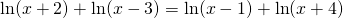### Solution

The equation is only defined when all logarithms are defined. This is the case when the following conditions are met: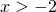and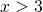and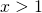and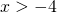thus if:We can write the equation as: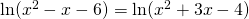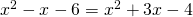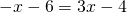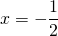This solution falls outside the interval given above and thus the equation has no solution.